# DeepLearning from scratch

Here is implementation of Neural Network from scratch without using any libraries of ML Only numpy is used for NN and matplotlib for plotting the results

## View on Github Page

visulization of deep layers are also shown in the examples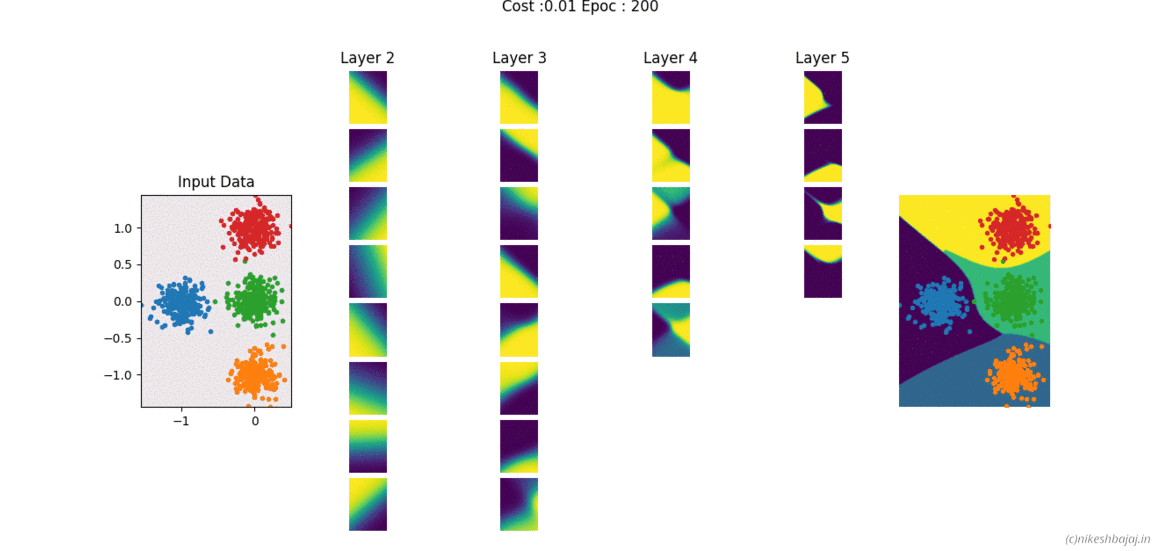## Implementation includes following

• Momentum
• RMSprop

### Regularization

• L2 Penalization
• Dropouts

### Activation functions

• Sigmoid, Tanh, ReLu, LeakyReLu, Softmax

### Data set

• Two class dataset : Gaussian, Linear, Moons, Spiral, Sinasodal
• Multiclass: gaussian distribuated data upto 9 classes

## for Two features, deep layers, decesion boundries and Learning curve can be visulize as shown in figures below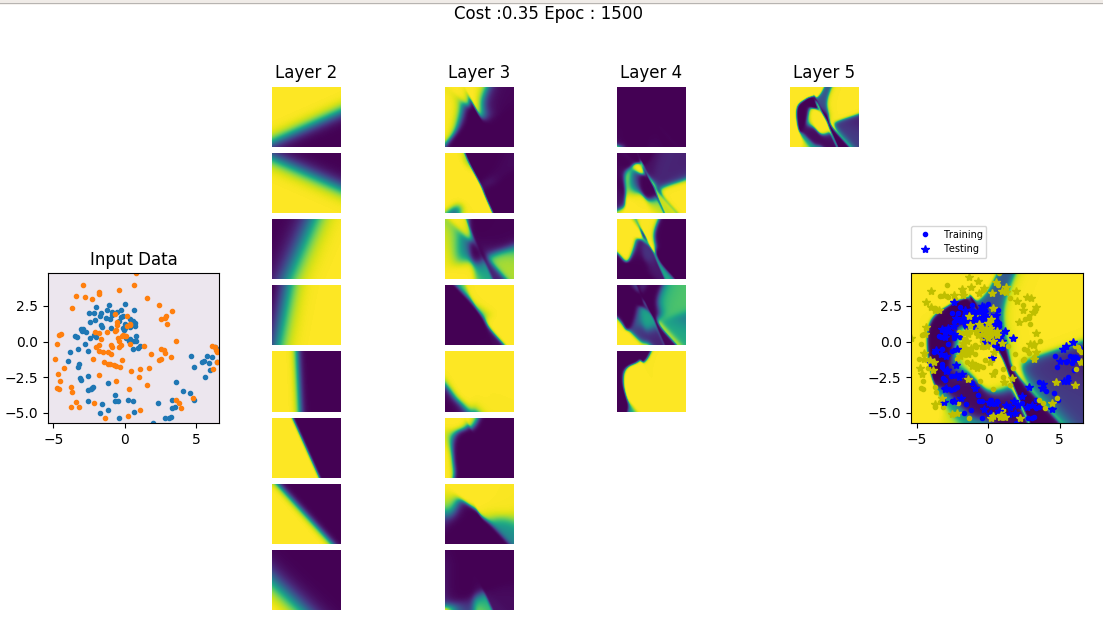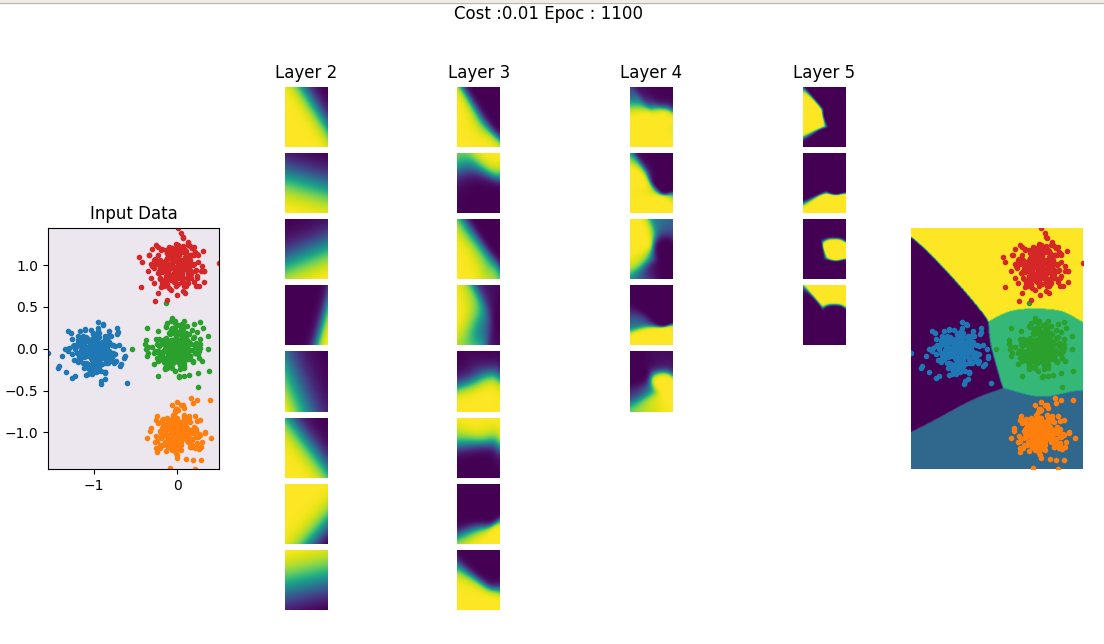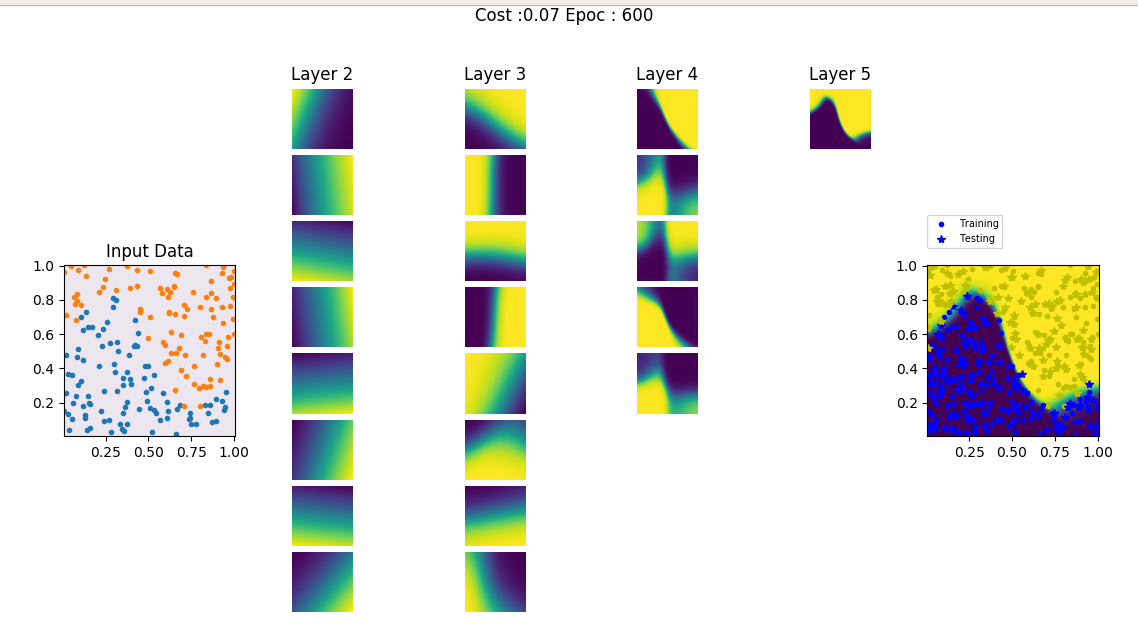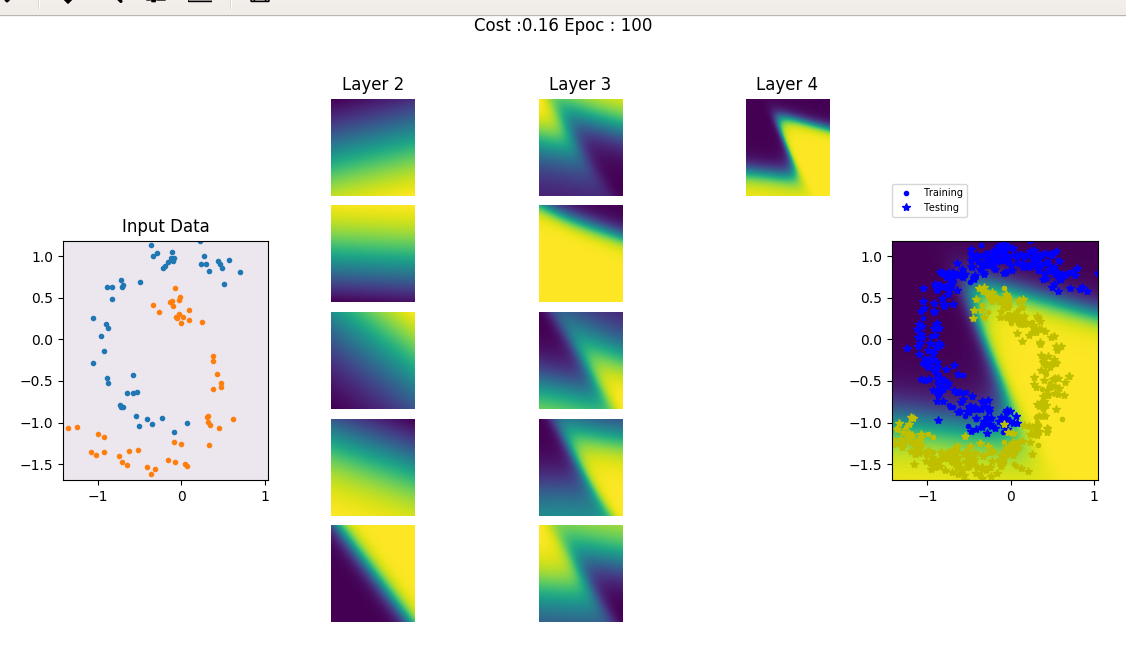## For more than two features, only Leaning curve is easy to plot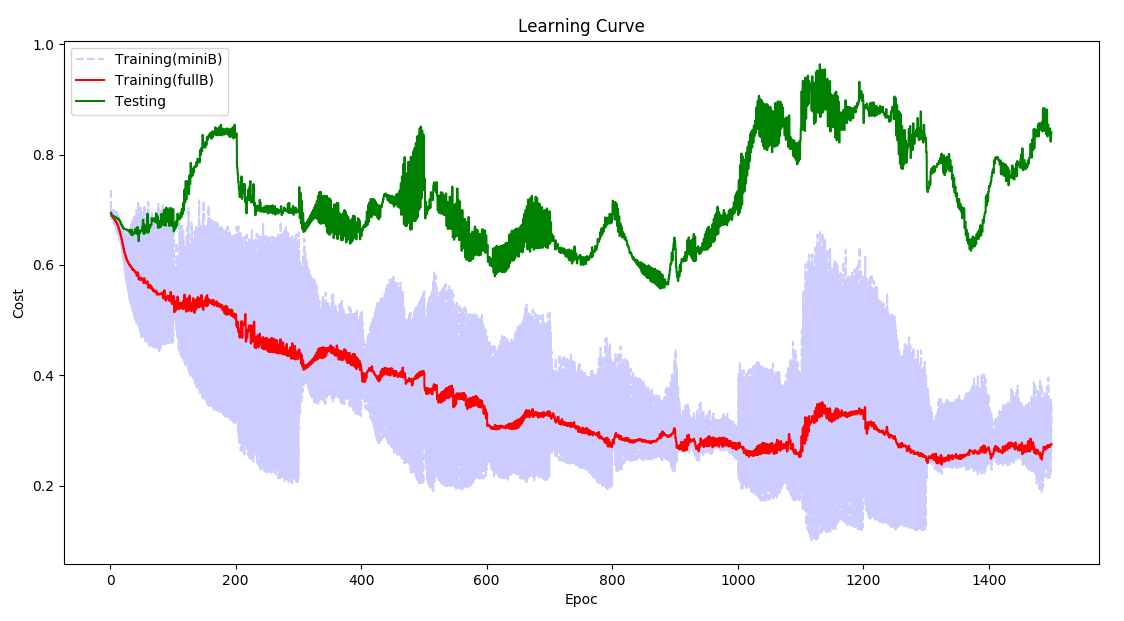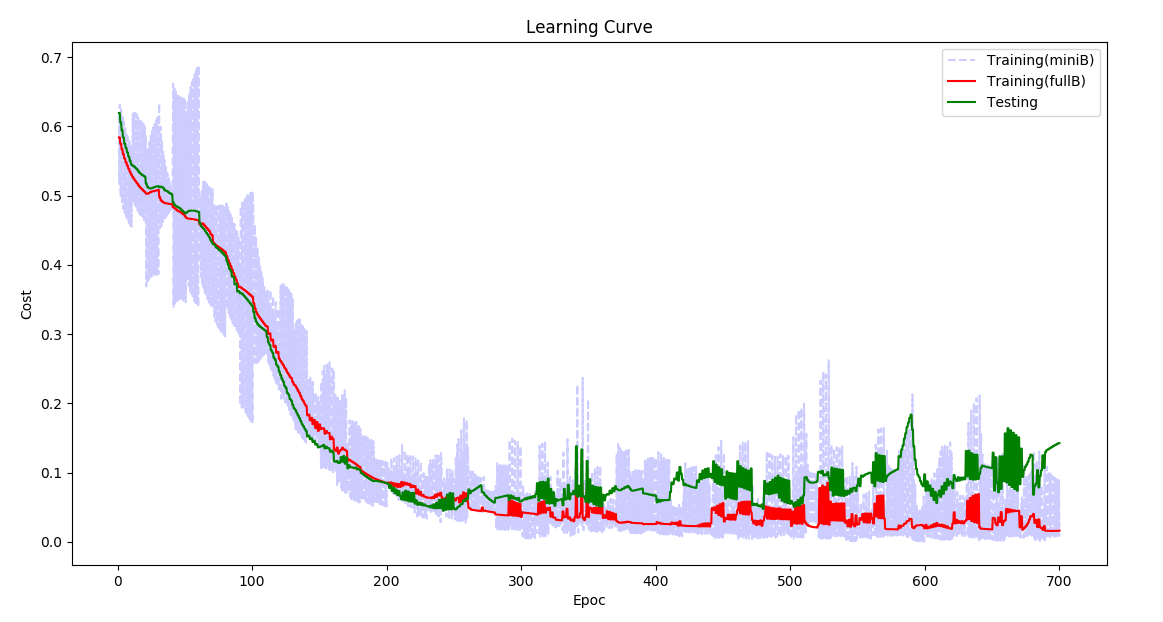## Instruction to run

### Requirements are only numpy and matplotlib

``````import numpy as np
import matplotlib.pyplot as plt
``````

### Import libraries

``````from DeepNet import deepNet
import DataSet as ds
``````

### Data

You can simulate the toy examples from DataSet library or use your own examples. Important is to remember for DeepNet, dimention of X should be (nf, N), where nf is number of features and N is numbe of examples.

#### Simulating data Xy from DataSet

``````X, y,_ = ds.create_dataset(N =200, Dtype = 'MOONS', noise=0.0,varargin = 'PRESET')
``````

Size of X will be =(2,200) and y =(1,200)

This will generate 200 samples for each class of Moons data with ‘PRESET’ arguements, you can add noise by fliping the classes of some example, noise takes fractional value to flips the class labels.

type `help(ds.create_dataset)` for more detail

### Neural Network

#### Size of Neural Network

``````Network =[3,3]

``````

Two hidden Layers with 3 neurons each, also can be `Network =[100, 50, 40, 200]` as deep as you like First and last layer of network will be decided based on dimention of X, and y. Size of first layer = number of features in X (=X.shape), Size of last layer will be 1 if there are two classes else equal to number of classes =(unique values in y)

#### Activation Functions

`NetAf = ['tanh','relu']`

first layer with tanh and next layer with relu activation function, if you pass only one then by defalut all the hidden layers will have same activation function. Other options for activation functions are Sigmoid `sig`, Leaky Rectifier Linear Unite `lrelu` By default if there are two classes, last layer activation function will be sigmoid for multiclass it will be softmax.

#### Learning rate

``````alpha=0.01
``````

#### Batch Size

``````miniBatchSize = 0.3
``````

this sets 30% as batch size, if `miniBatchSize = 1.0` then there will not be batch processing

#### Optimizer

``````AdamOpt=True
``````

if selected `AdamOpt=False` normal gradiet decent will be effective

#### Momentum Parameters

``````B1=0.9
B2=0.99
``````

These parameters can be tuned

#### L2 Regularizition

``````lambd =0.5
``````

if set to 0 no L2 regularization will be used

#### Dropouts

``````keepProb =[1.0, 0.8, 1.0]
``````

length of keepProb should be either 1 or eaual to number of layers if length of keepProb is 1 same probabilty of dropout will be used for all the layers expcept last layer.

Here is example to create Neural Network

``````NN = deepNet(X,y,Net = [3,3],NetAf =['tanh'], alpha=0.01,miniBatchSize = 0.3,
``````

### Training

``````NN.fit(itr=100)
``````

this allows you to train for 100 iteration and do any computation like checking cost, error, decesion boundries etc, as shown in example scripts. then for next 100 iteration just run `NN.fit(itr=100)`

### Priting Cost while training

`printCostAt =100` this will print cost after every 100 iterations. To disable set `printCostAt =-1` No cost will be printed then

### Testing Data (Optional)

Testing data can also to given to network, Network won’t use it for training, it will be only used for computing cost at every iteration for ploting Learning Curve and will also be shown on decesion boundries. By default Xts and yts are set to `None`

``````NN = deepNet(X,y,Xts =None, yts =None, ....
``````

### Predicting

``````yp, ypr = NN.predict(X)
``````

this will give you predicted class in yp and probabilities of all the classes in ypr

### Ploting Learning Curve

``````NN.PlotLCurve()
``````

### Ploting Decesion Boundries

This is only if X has two features `(X.shape==2)`

``````NN.PlotBoundries()
``````

### Plotting Hidden Layers for visulization of hidden low level features learned by Network

This is only if X has two features `(X.shape==2)`

``````NN.PlotBoundries(Layers=True)
``````

### Plotting learning curve and Decesion Boundries while training

``````fig1=plt.figure(1,figsize=(8,4))
fig2=plt.figure(2,figsize=(8,5))

for i in range(20):         ## 20 times
NN.fit(itr=10)          ## itr=10 iteretion each time
NN.PlotLCurve(pause=0)
fig1.canvas.draw()
NN.PlotBoundries(Layers=True,pause=0) # works only when there are two features (X.shape==2)
fig2.canvas.draw()

``````